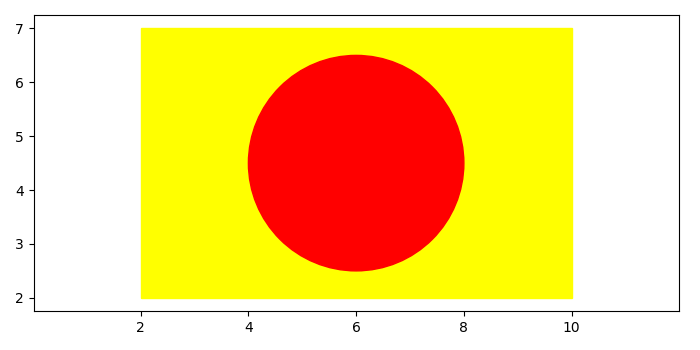# Plot a circle inside a rectangle in Matplotlib

To place a circle inside a rectangle, we can take the following steps −

• Create a new figure or activate an existing figure using figure() method.

• Add a subplot to the current axis.

• Create a rectangle and a circle instance.

• Add a rectangle patch to the current axis.

• Add a circle patch to the current axis.

• Scale x and y axes using xlim() and ylim() methods.

• To display the figure, use show() method.

## Example

import matplotlib
from matplotlib import pyplot as plt, patches
plt.rcParams["figure.figsize"] = [7.00, 3.50]
plt.rcParams["figure.autolayout"] = True
fig = plt.figure()
rect = patches.Rectangle((2, 2), 8, 5, color='yellow')
circle = patches.Circle((6, 4.5), radius=2, color='red')
plt.show()